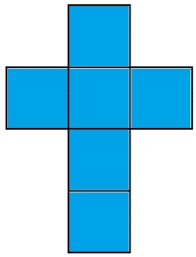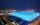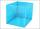# Length of the edge

Find the length of the edge of a cube with a cm2 surface and a volume in cm3 expressed by the same number.

a =  6 cm

### Step-by-step explanation:Did you find an error or inaccuracy? Feel free to write us. Thank you!Tips to related online calculators
Tip: Our volume units converter will help you with the conversion of volume units.

## Related math problems and questions:

• The cubeThe cube has a surface area of 216 dm2. Calculate: a) the content of one wall, b) edge length, c) cube volume.
• Cube surface and volumeFind the surface of the cube with a volume of 27 dm3.
• Area to volumeIf the surface area of a cube is 486, find its volume.
• Cube surfce2volumeCalculate the volume of the cube if its surface is 150 cm2.
• Volume and areaWhat is the volume of a cube which has an area of 361 cm2?
• TerezaThe cube has an area of base 256 mm2. Calculate the edge length, volume, and area of its surface.
• Cube 8The surface of the cube is 0.54 m2. Calculate the length of the cube edge.
• Cube wallThe surface of the first cube wall is 64 m2. The second cube area is 40% of the surface of the first cube. Determine the length of the edge of the second cube (x).
• Cube surface and volumeThe surface of the cube is 500 cm2, how much cm3 will be its volume?
• The cubeThe cube has a surface of 600 cm2. What is its volume?
• Cube V2SThe volume of the cube is 27 dm cubic. Calculate the surface of the cube.
• Cube edgeDetermine the edges of the cube when the surface is equal to 37.5 cm square.
• The cubeThe cube has a surface area of 486 m ^ 2. Calculate its volume.
• Cube 6The surface area of one wall cube is 1600 cm square. How many liters of water can fit into the cube?
• Cube 5The surface of the cube is 15.36 dm2. How will change the surface area of this cube if the length of the edges is reduced by 2 cm?
• Cube into cylinderIf we dip a wooden cube into a barrel with a 40cm radius, the water will rise 10 cm. What is the size of the cube edge?
• Two rectangular boxesTwo rectangular boxes with dimensions of 5 cm, 8 cm, 10 cm, and 5 cm, 12 cm, 1 dm are to be replaced by a single cube box of the same cubic volume. Calculate its surface.